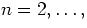3.2.1.1 Required Keywords

The keywords required for an ordinary array are also required for random groups, in the same order.

1.
SIMPLE (logical) ``T'' indicates a file in conformance to standard FITS.
2.
BITPIX (integer) describes the representation of the values of the individual arrays and of the parameters. The parameters must be of the same data type as the array members. The values have the same meaning as for Basic FITS.

3.
NAXIS (integer) is one more than the number of axes in each array (because NAXIS1 is used as a groups indicator instead of an actual axis). The largest possible value is 999, representing 998 axes.

4.
NAXIS1 (integer) is set to 0 to indicate that no primary data array follows the primary header.

5.
NAXISn,NAXIS (integer) is the number of elements along axis n-1 of the array. The index varies most rapidly along the n=2 axis, least rapidly along the n=NAXIS axis.

There must be no other keywords between the ones listed above.

Additional keywords follow, which must include among them

• GROUPS (logical) must have the value ``T'', to indicate that the data records following the primary data array are random groups.
• PCOUNT (integer) is the number of parameters preceding each data array.
• GCOUNT (integer) is the number of groups (parameters + array) in the random groups records.
• END (no value) is the last keyword. The header is filled with ASCII blanks.

The PCOUNT and GCOUNT keywords tell the reader the number NBITS of bits in the random groups records exclusive of fill:

 NBITS = ABS(BITPIX) × GCOUNT × (PCOUNT + NAXIS × NAXIS3 × ... × NAXISm), (3.7)

where m is the value of NAXIS.